• 论文趋势分析（python+excel+tableau）1数据读取1.1读取原始数据1.2抽取5%的数据作为样本进行分析1.3 爬取论文类别信息1.4处理多种类论文1.5表连接1.6提取论文的发表年份和月份1.7提取论文的页数，图数1.8提取论文的...

本文github地址：链接
论文趋势分析专栏：链接
本文被DataWhale公众号转发：链接

论文趋势分析（python+excel+tableau）1数据读取1.1读取原始数据1.2抽取5%的数据作为样本进行分析1.3 爬取论文类别信息1.4处理多种类论文1.5表连接1.6提取论文的发表年份和月份1.7提取论文的页数，图数1.8提取论文的作者数量1.9删除重复的论文2论文趋势分析-python2.1论文大类总体分析2.1.1各大类论文总数2.1.2各类论文数量随年份的变化2.1.3论文总数量随时间的变化2.1.4不同大类论文页数的不同2.1.5不同大类合作作者数量的不同2.2计算机领域论文趋势分析2.2.1各领域论文的总数量2.2.2各领域论文数量随时间的变换2.2.3论文页数2.2.4计算机视觉领域的合作作者数量最多3使用Excel分析3.1创建数据透视表3.2不同大类的论文数量随时间的变化趋势4使用tableau进行分析4.1计算机领域论文数量差异4.2计算机领域论文数量变化（top5)4.3计算机领域论文页数差异总结
import pandas as pd
import numpy as np
import seaborn as sns
import matplotlib.pyplot as plt
import json

from bs4 import BeautifulSoup #用于爬取arxiv的数据
import re #用于正则表达式，匹配字符串的模式
import requests #用于网络连接，发送网络请求，使用域名获取对应信息

1数据读取
1.1读取原始数据

数据集来源：数据集链接；
数据集的格式如下：

id：arXiv ID，可用于访问论文；
submitter：论文提交者；
authors：论文作者；
title：论文标题；
comments：论文页数和图表等其他信息；
journal-ref：论文发表的期刊的信息；
doi：数字对象标识符，https://www.doi.org；
report-no：报告编号；
categories：论文在 arXiv 系统的所属类别或标签；
license：文章的许可证；
abstract：论文摘要；
versions：论文版本；
authors_parsed：作者的信息。

# 读取json数据
def readArxivFile(path, columns=['id', 'submitter', 'authors', 'title', 'comments', 'journal-ref', 'doi','report-no', 'categories', 'license', 'abstract', 'versions','update_date', 'authors_parsed'], count=None):
data  = []
with open(path, 'r') as f:
for idx, line in enumerate(f):
if idx == count:
break

d = json.loads(line)
d = {col : d[col] for col in columns}
data.append(d)

data = pd.DataFrame(data)
return data

data = readArxivFile('D:\code\Github\data\AcademicTrendsAnalysis/arxiv-metadata-oai-snapshot.json', ['id', 'submitter', 'authors', 'title', 'comments', 'journal-ref', 'categories', 'abstract', 'versions','update_date', 'authors_parsed'])

1.2抽取5%的数据作为样本进行分析

注释掉此段代码，就可以对全部数据进行分析，限于本人的机子性能有限，所以只取5%的数据进行分析

data = data.sample(frac =0.05,replace = False,random_state = 1 )
# 清理内存垃圾
import gc
gc.collect()

76

# 存储转换后的数据
data.to_csv('D:\code\Github\data\AcademicTrendsAnalysis\data.csv',index = False)

data.head(3)


id
submitter
authors
title
comments
journal-ref
categories
abstract
versions
update_date
authors_parsed

0
1506.04017
Serena Ng
Jean-Jacques Forneron and Serena Ng
A Likelihood-Free Reverse Sampler of the Poste...
NaN
NaN
stat.ME
This paper considers properties of an optimi...
[{'version': 'v1', 'created': 'Fri, 12 Jun 201...
2015-12-02
[['Forneron', 'Jean-Jacques', ''], ['Ng', 'Ser...

1
gr-qc/9211024
NaN
R. Mansouri and M.Mohazzab (BROWN)
Tunneling in Anisotropic Cosmological Models
13 pages, phyzzx
Class.Quant.Grav.10:1353-1359,1993
gr-qc
Tunneling rate is investigated in homogenous...
[{'version': 'v1', 'created': 'Thu, 19 Nov 199...
2010-04-06
[['Mansouri', 'R.', '', 'BROWN'], ['Mohazzab',...

2
2011.02152
Rotem Liss
Rotem Liss, Tal Mor
From Practice to Theory: The "Bright Illuminat...
17 pages
NaN
quant-ph cs.CR
The "Bright Illumination" attack [Lydersen e...
[{'version': 'v1', 'created': 'Wed, 4 Nov 2020...
2020-11-05
[['Liss', 'Rotem', ''], ['Mor', 'Tal', '']]

1.3 爬取论文类别信息

group_name 论文大类
archive_name 论文子类（除了物理学领域，其他种类都没有子类）
archive_id 论文子类的缩写
category_name 论文细类的名称
categories 论文细类的缩写

#爬取所有的类别
website_url = requests.get('https://arxiv.org/category_taxonomy').text #获取网页的文本数据
soup = BeautifulSoup(website_url,'lxml') #爬取数据，这里使用lxml的解析器，加速
root = soup.find('div',{'id':'category_taxonomy_list'}) #找出 BeautifulSoup 对应的标签入口
tags = root.find_all(["h2","h3","h4","p"], recursive=True) #读取 tags

#初始化 str 和 list 变量
level_1_name = ""
level_2_name = ""
level_2_code = ""
level_1_names = []
level_2_codes = []
level_2_names = []
level_3_codes = []
level_3_names = []
level_3_notes = []

#进行
for t in tags:
if t.name == "h2":
level_1_name = t.text
level_2_code = t.text
level_2_name = t.text
elif t.name == "h3":
raw = t.text
level_2_code = re.sub(r"(.*)$$(.*)$$",r"\2",raw) #正则表达式：模式字符串：(.*)$$(.*)$$；被替换字符串"\2"；被处理字符串：raw
level_2_name = re.sub(r"(.*)$$(.*)$$",r"\1",raw)
elif t.name == "h4":
raw = t.text
level_3_code = re.sub(r"(.*) $$(.*)$$",r"\1",raw)
level_3_name = re.sub(r"(.*) $$(.*)$$",r"\2",raw)
elif t.name == "p":
notes = t.text
level_1_names.append(level_1_name)
level_2_names.append(level_2_name)
level_2_codes.append(level_2_code)
level_3_names.append(level_3_name)
level_3_codes.append(level_3_code)
level_3_notes.append(notes)

#根据以上信息生成dataframe格式的数据
df_taxonomy = pd.DataFrame({
'group_name' : level_1_names,
'archive_name' : level_2_names,
'archive_id' : level_2_codes,
'category_name' : level_3_names,
'categories' : level_3_codes,
'category_description': level_3_notes

})
df_taxonomy.head()

# 存储论文类别信息
df_taxonomy.to_csv('D:\code\Github\data\AcademicTrendsAnalysis\categories.csv',index = False)

data = pd.read_csv('D:\code\Github\data\AcademicTrendsAnalysis\data.csv')
df_taxonomy = pd.read_csv('D:\code\Github\data\AcademicTrendsAnalysis\categories.csv')

df_taxonomy.head(3)


group_name
archive_name
archive_id
category_name
categories
category_description

0
Computer Science
Computer Science
Computer Science
Artificial Intelligence
cs.AI
Covers all areas of AI except Vision, Robotics...

1
Computer Science
Computer Science
Computer Science
Hardware Architecture
cs.AR
Covers systems organization and hardware archi...

2
Computer Science
Computer Science
Computer Science
Computational Complexity
cs.CC
Covers models of computation, complexity class...

1.4处理多种类论文

有的论文同时属于多个种类，在统计时，只按照其最重要的类别（第一个类别）

data.categories.iloc[:5]

0                            stat.ME
1                              gr-qc
2                     quant-ph cs.CR
3                  cond-mat.mtrl-sci
4    math.FA math.AP math.CA math.DG
Name: categories, dtype: object

看到类别数据以空格分割
data['category'] = data.categories.str.split(' ',expand=True)
print(data['category'].nunique())

172

1.5表连接
data = data.merge(df_taxonomy,how='left',left_on='category',right_on='categories')

data.shape

(89846, 18)

1.6提取论文的发表年份和月份
data['month'] = pd.to_datetime(data.update_date).dt.month
data['year'] = pd.to_datetime(data.update_date).dt.year

1.7提取论文的页数，图数
# 将评论转换成pd.string类型
data.comments = data.comments.astype('string')
pat = '(\d+) pages'
data['pages'] = data.comments.str.extract(pat=pat)

pat = '(\d+) figures'
data['figure'] = data.comments.str.extract(pat)

# 将文本转换为数字
data.pages = data.pages.fillna('0').astype(int)
# 避免0值参与计算的影响，将0值转换为空值，聚合计算时会自动忽略
data.loc[data.pages == 0] = np.nan

# 将文本转换为数字
data.figure = data.figure.fillna('0').astype(int)
# 避免0值参与计算的影响，将0值转换为空值，聚合计算时会自动忽略
data.figure.loc[data.figure == 0] = np.nan

1.8提取论文的作者数量

将文本型的列表转换为python列表

import ast
data.authors_parsed =  data.authors_parsed.apply(ast.literal_eval)


统计作者数量

data['author_num'] = data.authors_parsed.apply(len)

1.9删除重复的论文

一篇论文可能多次提交，因此有多个版本，只保留第一个版本

data = data.drop_duplicates(['id','category'],keep = 'first')

data.to_csv('D:\code\Github\data\AcademicTrendsAnalysis\data_processed.csv',index=False)

2论文趋势分析-python
2.1论文大类总体分析
2.1.1各大类论文总数

物理，数学，计算机科学是数量最多的三大门类
统计学，计量生物学等门类的数量较少

group1 = data.groupby('group_name')['id'].agg('count').sort_values(ascending = False).to_frame()
group1 = group1.reset_index()
group1.columns = ['group_name','count']

sns.barplot(x = 'count',y = 'group_name',data = group1)

<matplotlib.axes._subplots.AxesSubplot at 0x1ca624ed730>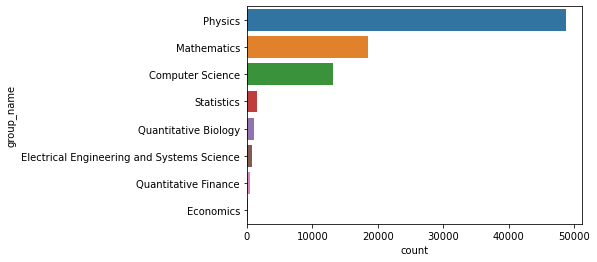2.1.2各类论文数量随年份的变化

可以看到计算机科学的论文数量随着时间，增长越来越快，数学次之
物理学领域的论文数量变化较大，但总体也呈现上升趋势
其他领域的论文数量则增幅很小

group2 = data.groupby(['group_name','year'])['id'].agg('count').to_frame()
group2 = group2.reset_index()
group2.columns = ['group_name','year','count']

sns.relplot(data=group2,x='year',y = 'count',hue='group_name',kind='line')

<seaborn.axisgrid.FacetGrid at 0x1ca63d96130>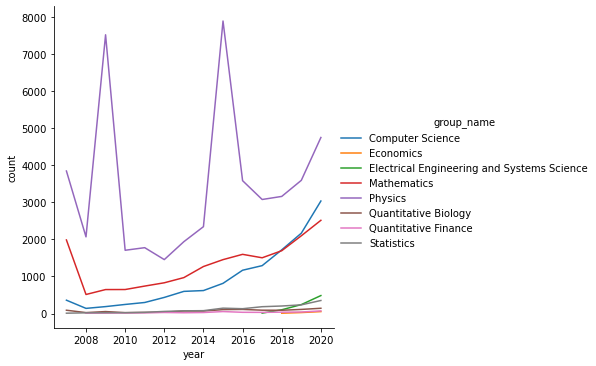sns.catplot(data = group2,x = 'year',y ='count',col='group_name'
,col_wrap=2,height=6,aspect=1.2,kind='bar')

<seaborn.axisgrid.FacetGrid at 0x1ca62d0baf0>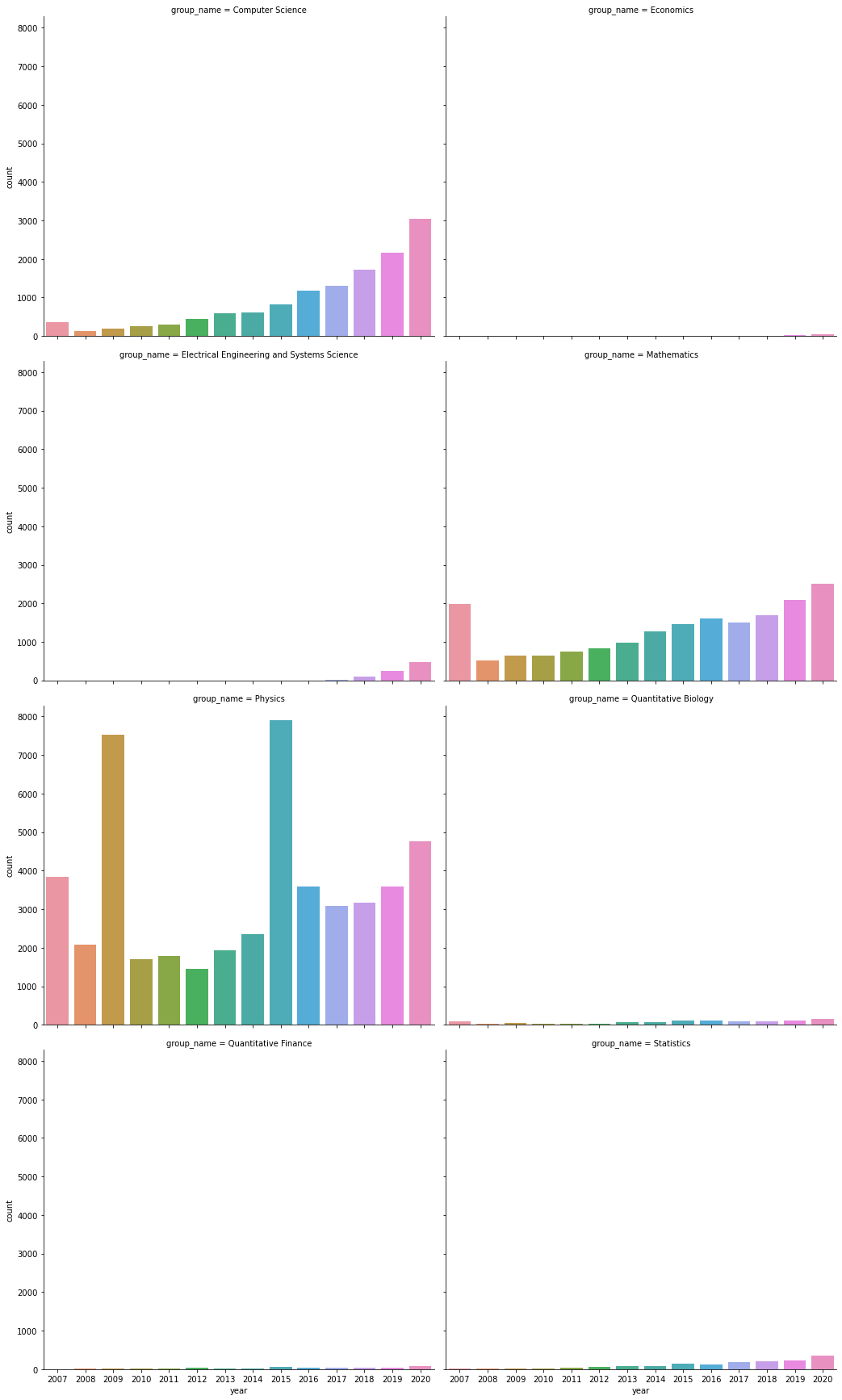2.1.3论文总数量随时间的变化
group3 = data.groupby('year')['id'].agg('count').sort_values(ascending = False).to_frame().reset_index()
group3.columns = ['year','count']
plt.figure(figsize = (10,8))
sns.barplot(data=group3,x='year',y='count')

<matplotlib.axes._subplots.AxesSubplot at 0x1ca65815940>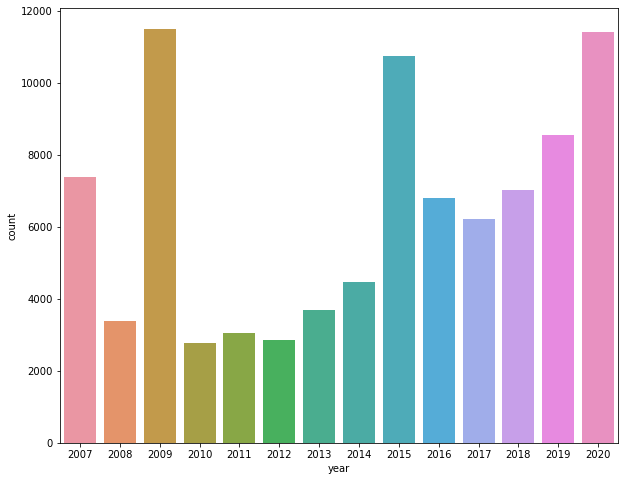2.1.4不同大类论文页数的不同

可以看到计算机科学的平均论文页数并不算高
经济学，数学，统计学的论文页数较多

group4 = data.groupby('group_name')['pages'].agg('mean').sort_values(ascending = False).to_frame().reset_index()
group4.columns = ['group_name','pages']
sns.barplot(data=group4,x='pages',y='group_name')

<matplotlib.axes._subplots.AxesSubplot at 0x1ca6c4183a0>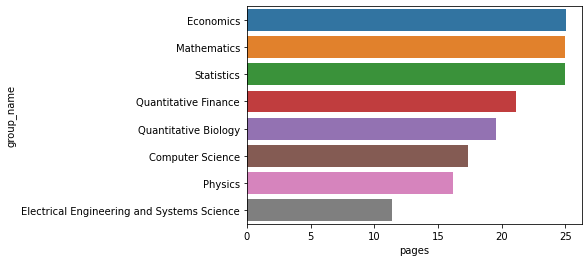2.1.5不同大类合作作者数量的不同

物理学领域论文平均每篇的作者数量最多，为5人
数学领域论文平均每篇的作者数量最少，只有不到2人

group4 = data.groupby('group_name')['author_num'].agg('mean').sort_values(ascending = False).to_frame().reset_index()
group4.columns = ['group_name','author_num']
sns.barplot(data=group4,x='author_num',y='group_name')

<matplotlib.axes._subplots.AxesSubplot at 0x1ca6ba9faf0>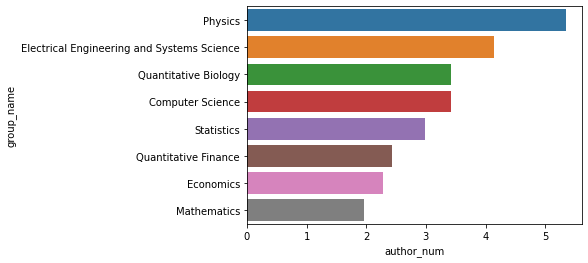2.2计算机领域论文趋势分析
2.2.1各领域论文的总数量

计算机视觉，机器学习果然是计算机科学论文最多的领域

group5 = data.loc[data.group_name == 'Computer Science'].groupby('category_name')['id'].agg('count').sort_values(ascending = False).to_frame().reset_index()
group5.columns = ['category_name','count']
plt.figure(figsize=(12,10))
sns.barplot(data=group5,x='count',y='category_name')

<matplotlib.axes._subplots.AxesSubplot at 0x1ca7165a1f0>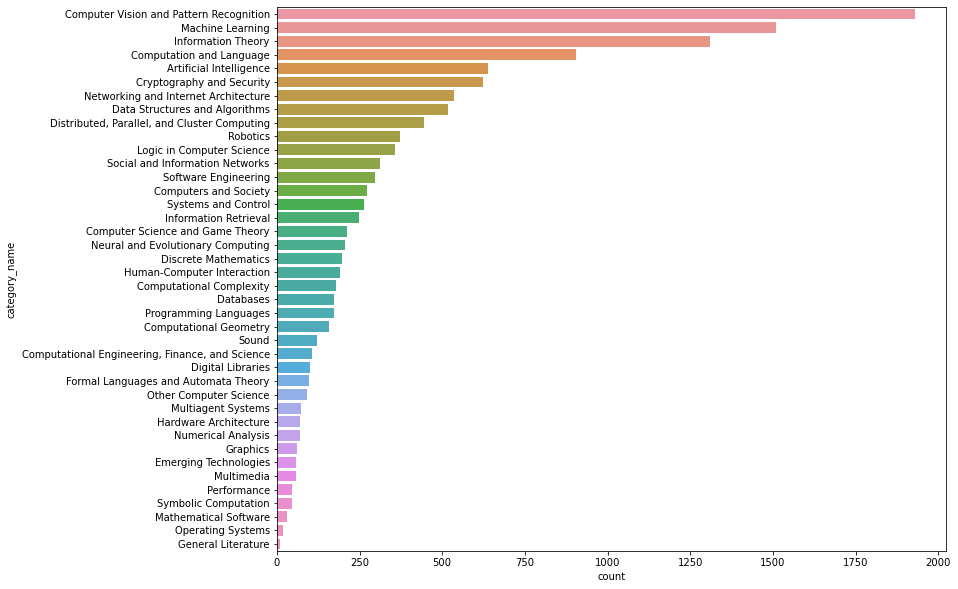2.2.2各领域论文数量随时间的变换

可以看到除了机器学习，机器视觉，自然语言处理增长较快之外，其他领域的论文数量增长都比较慢
信息理论，密码学与安全，机器人是除了ML，CV，NLP之外发表论文数量比较多的领域
ML和CV还是计算机科学领域最火的方向

group6 = data.loc[data.group_name == 'Computer Science'].groupby(['category_name','year'])['id'].agg('count').sort_values(ascending = False).to_frame().reset_index()
group6.columns = ['category_name','year','count']
sns.relplot(data=group6,x='year',y = 'count',hue='category_name',kind='line',height = 10,style = 'category_name',palette='husl')

<seaborn.axisgrid.FacetGrid at 0x1ca6c6c5070>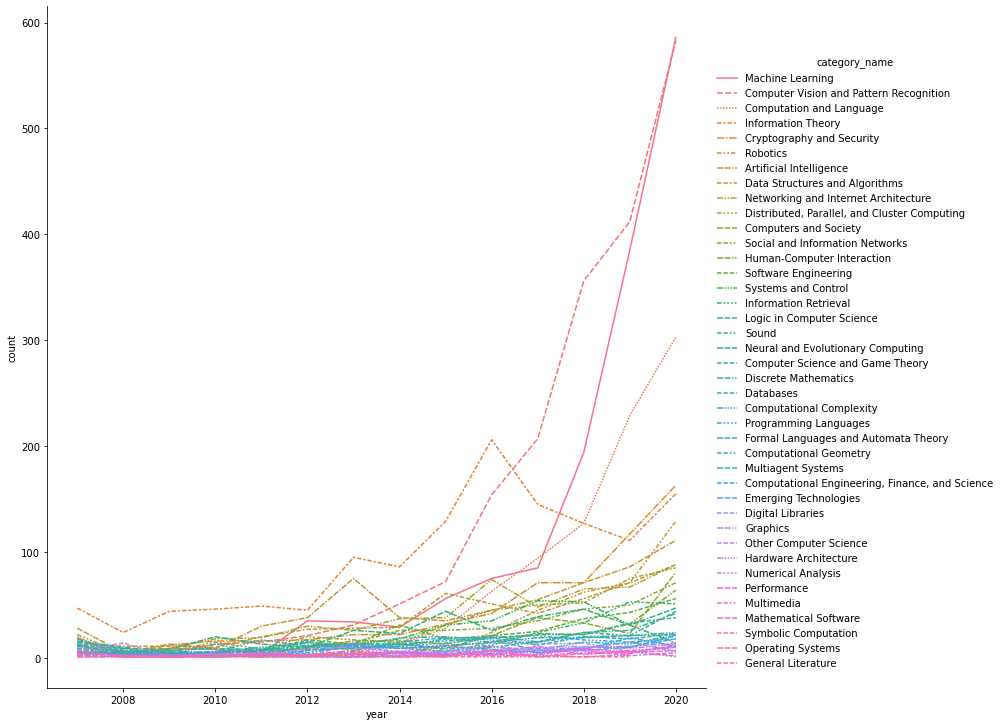Y轴相同刻度，查看相对数量

sns.catplot(data = group6,x = 'year',y ='count',col='category_name'
,col_wrap=4,height=5,aspect=1.2,kind='bar')

<seaborn.axisgrid.FacetGrid at 0x1ca6c2eb7f0>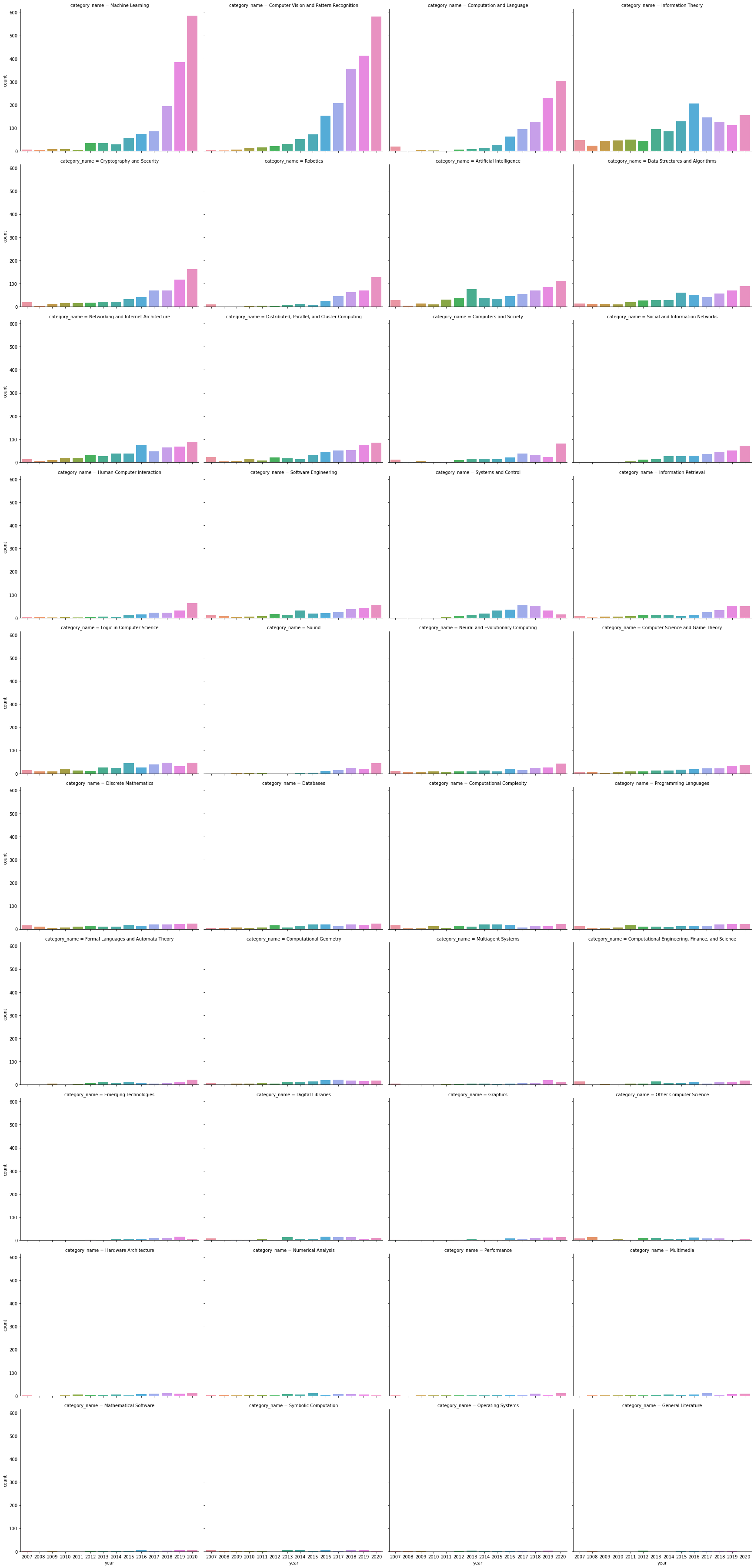Y轴不同刻度，查看变化趋势

sns.catplot(data = group6,x = 'year',y ='count',col='category_name'
,col_wrap=4,height=5,aspect=1.2,kind='bar',sharey=False)

<seaborn.axisgrid.FacetGrid at 0x1ca6c474130>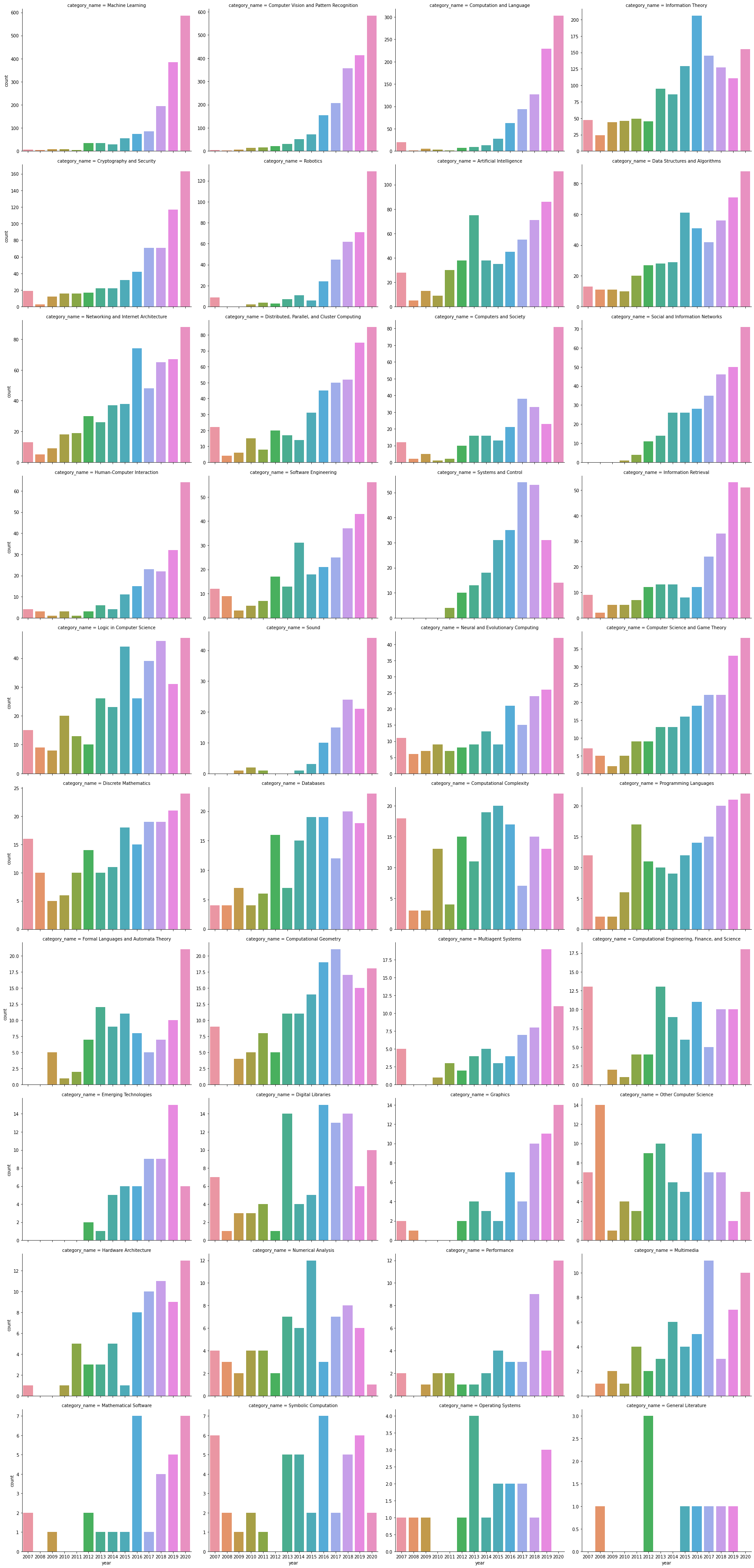2.2.3论文页数

人工智能，编程语言，数值分析领域的论文页数最长
CV和NLP的平均页数未进入前20

group7 = data.loc[data.group_name == 'Computer Science'].groupby('category_name')['pages'].agg('mean').sort_values(ascending = False).to_frame().reset_index().head(20)
group7.columns = ['category_name','mean_pages']
plt.figure(figsize=(6,8))
sns.barplot(data=group7,x='mean_pages',y='category_name')

<matplotlib.axes._subplots.AxesSubplot at 0x1ca76e637f0>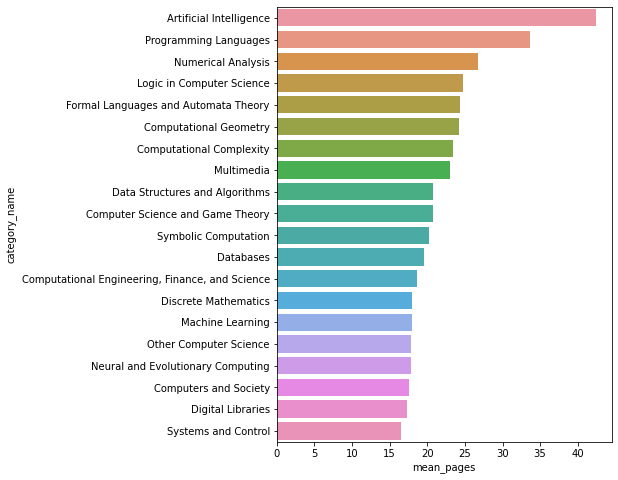2.2.4计算机视觉领域的合作作者数量最多

group8 = data.loc[data.group_name == 'Computer Science'].groupby('category_name')['author_num'].agg('mean').sort_values(ascending = False).to_frame().reset_index().head(20)
group8.columns = ['category_name','mean_author_num']
plt.figure(figsize=(6,8))
sns.barplot(data=group8,x='mean_author_num',y='category_name')

<matplotlib.axes._subplots.AxesSubplot at 0x1ca772646d0>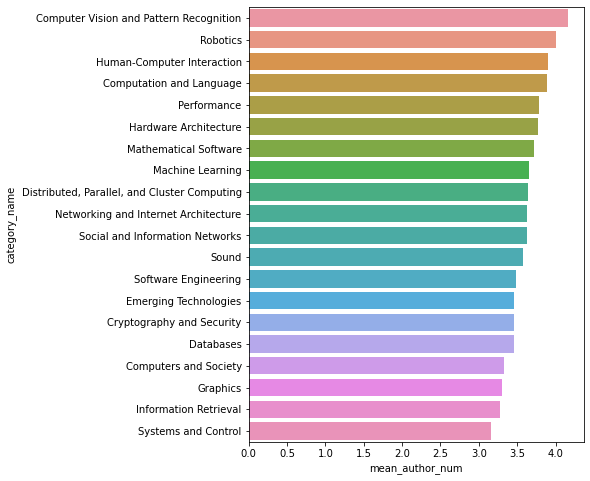3使用Excel分析
3.1创建数据透视表

将存储的csv文件另存为excel文件，并随便创建打开一个excel文件
点击数据选项卡-获取外部数据-现有连接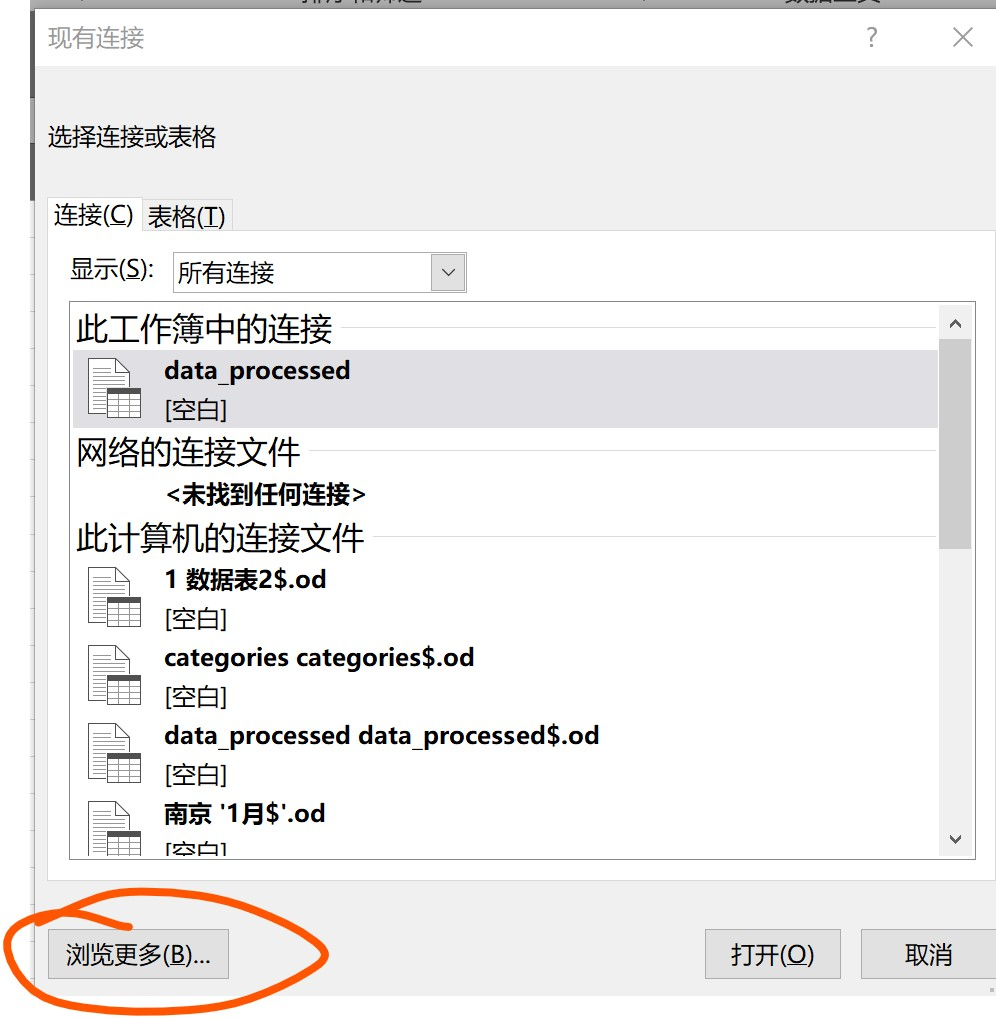点击浏览，选择上面保存的data_processed.xlsx文件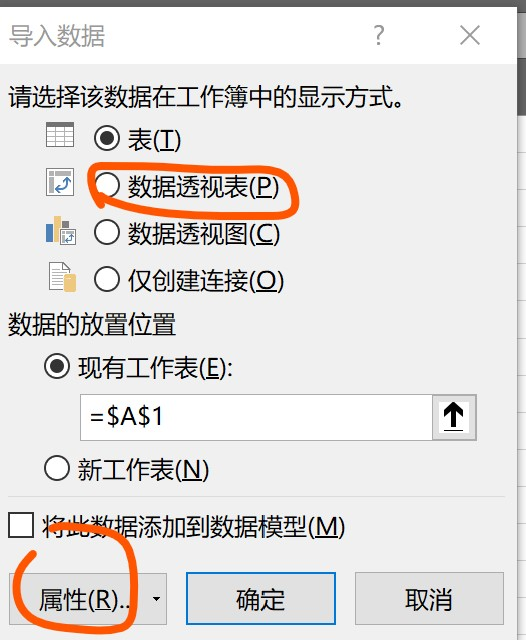更改属性，输入sql语句：

	select * from [D:\code\Github\data\AcademicTrendsAnalysis\data_processed.xlsx].[data_processed$]A left join [D:\code\Github\data\AcademicTrendsAnalysis\categories.xlsx].[categories$]B on A.category = B.categories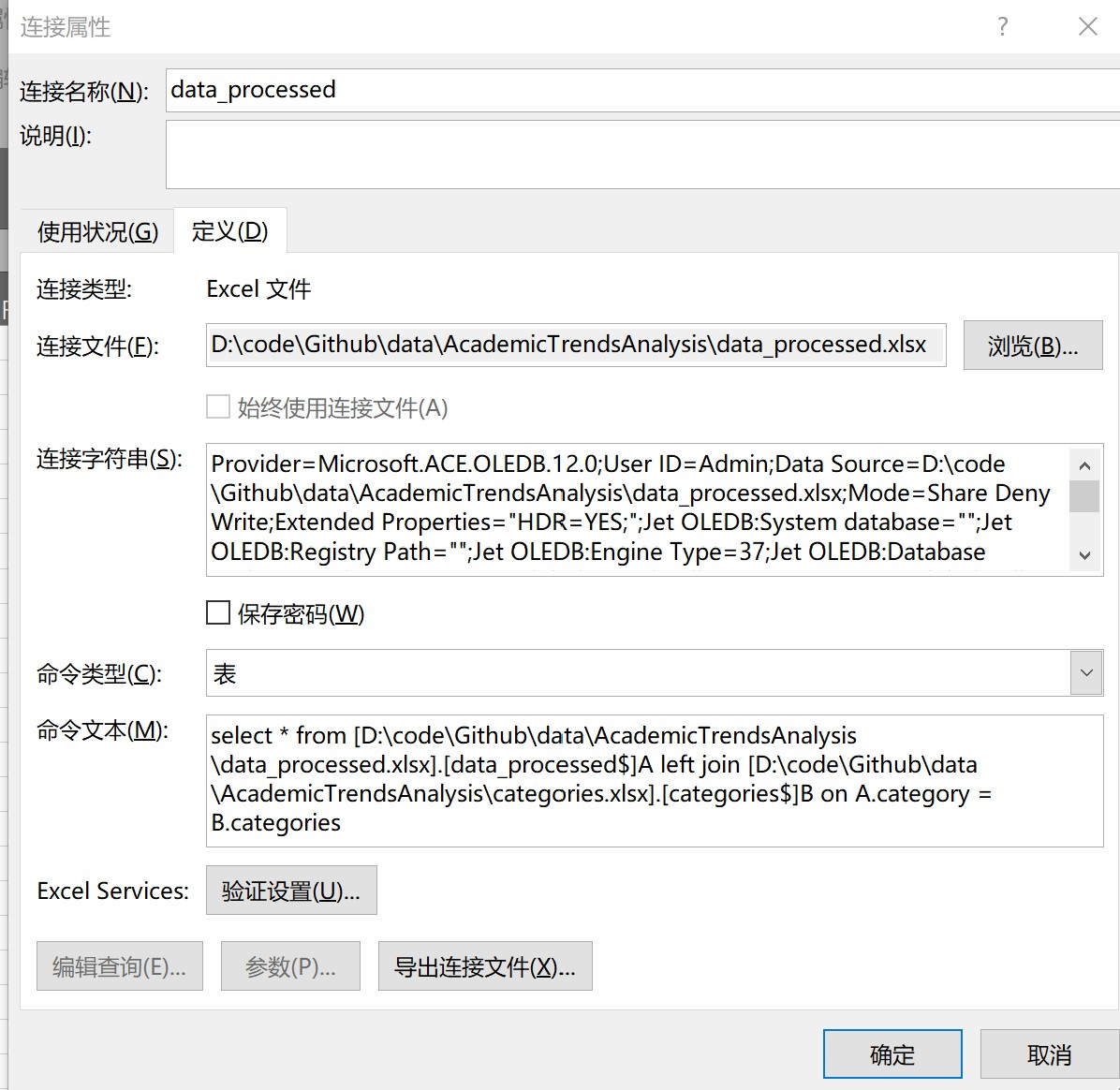5.  点击确定，创建数据透视表
3.2不同大类的论文数量随时间的变化趋势

在数据透视表中选择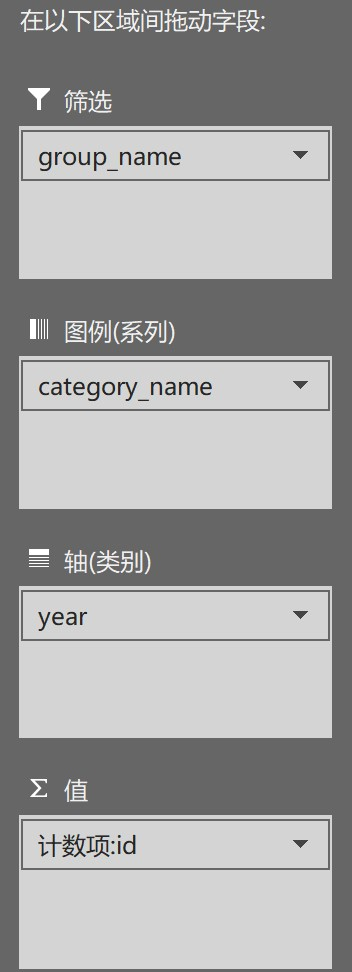2. 插入切片器，和折线图（前10）
计算机领域的论文变化趋势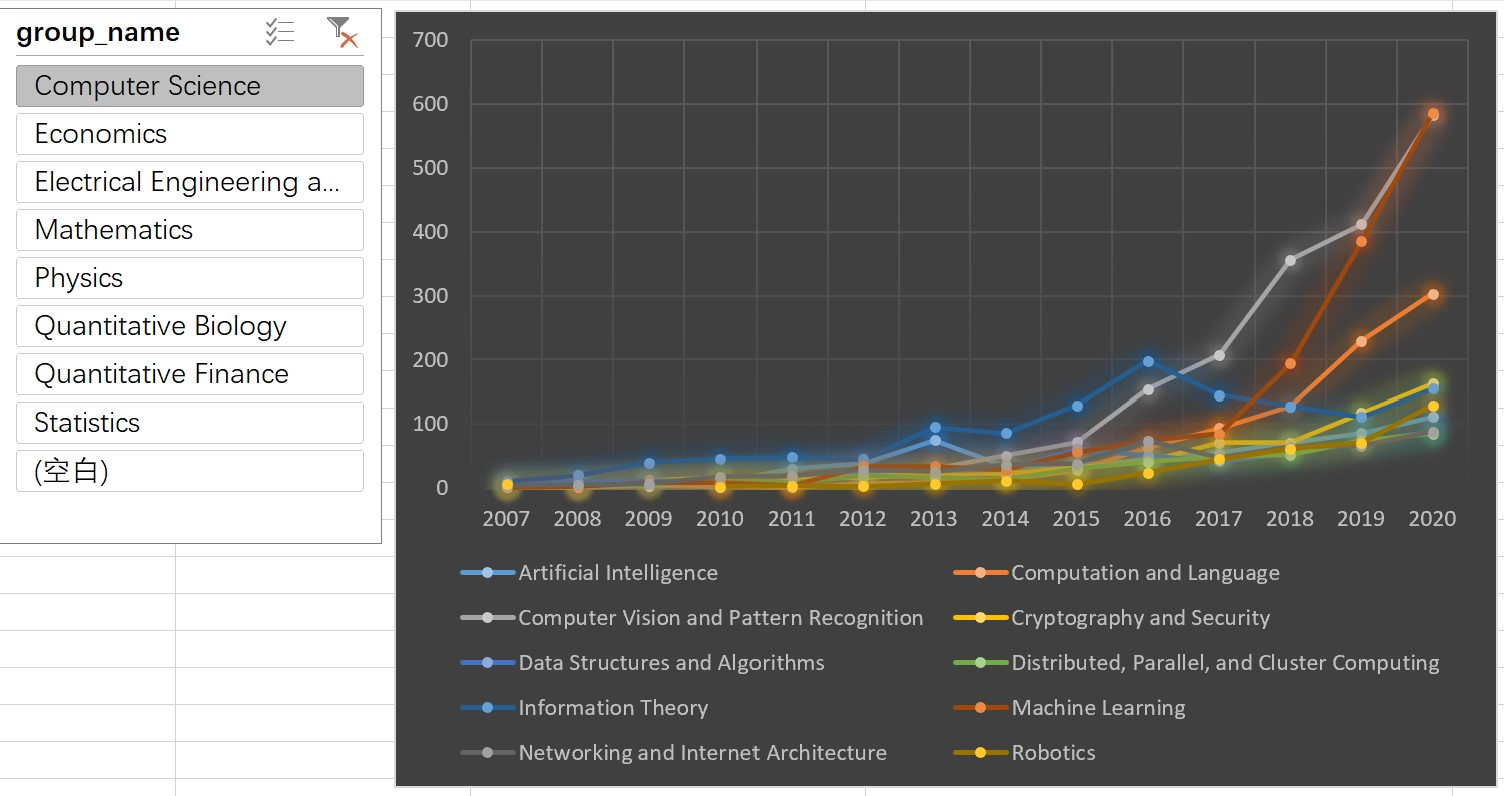更改切片器选择，物理学领域的论文趋势变化（前10）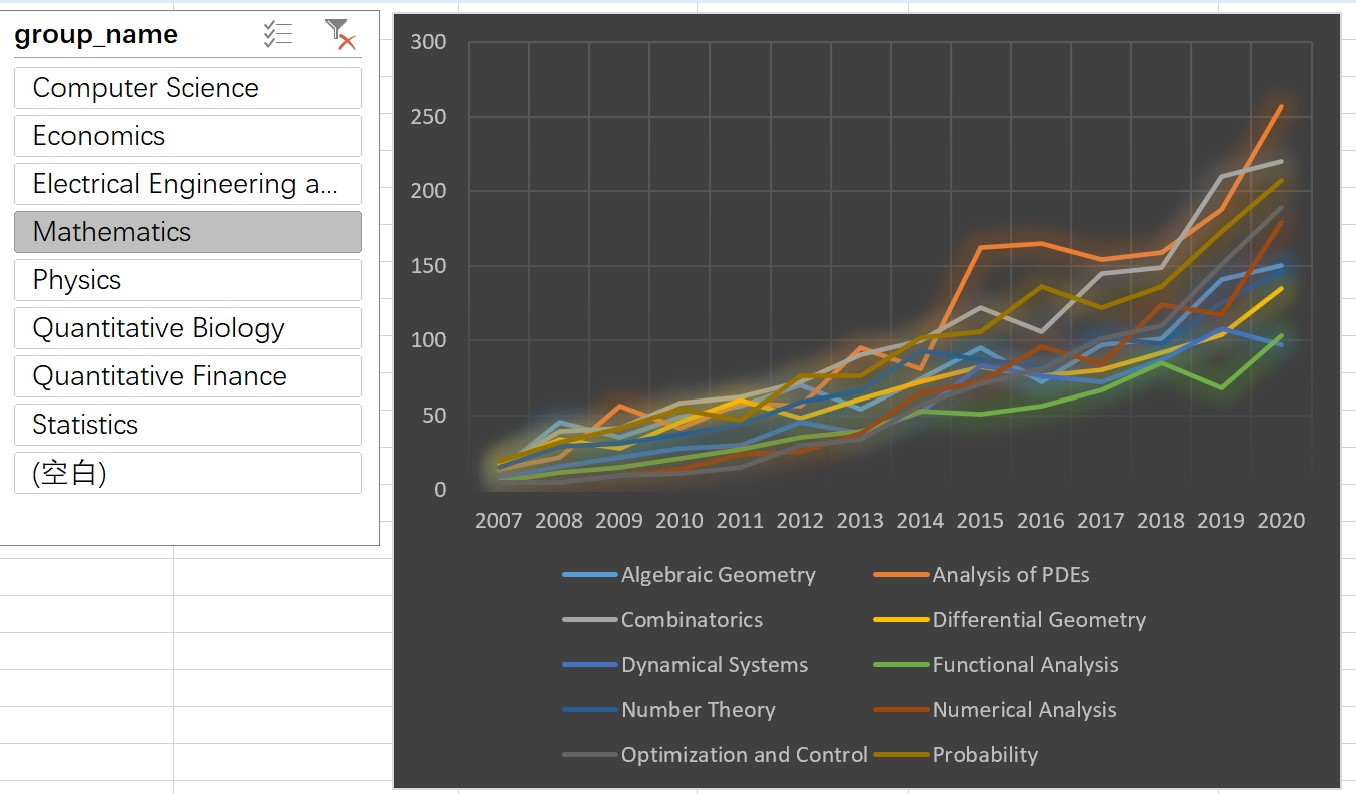3. 更改透视表设计，可以实现python的全部分析结果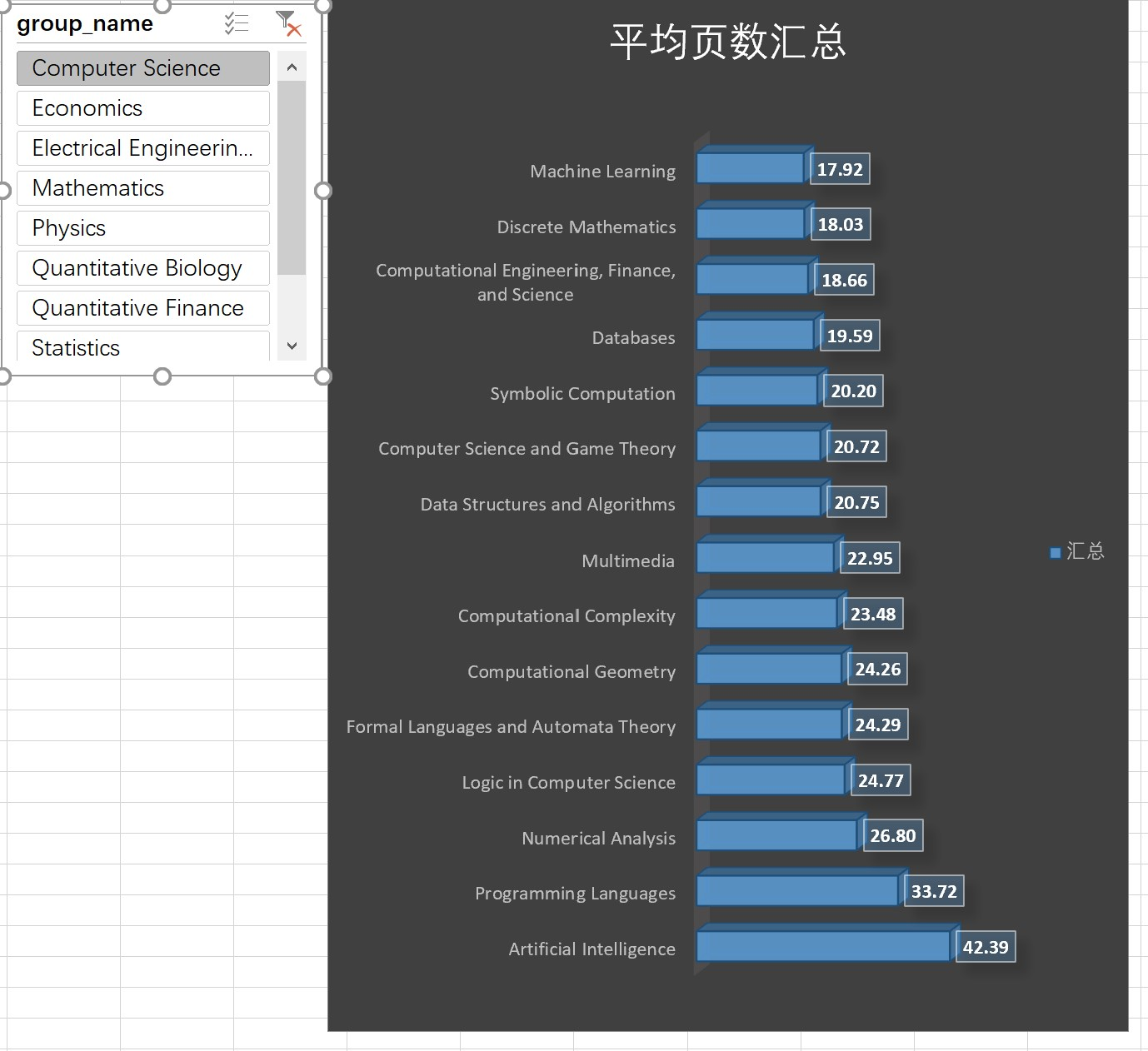4使用tableau进行分析
4.1计算机领域论文数量差异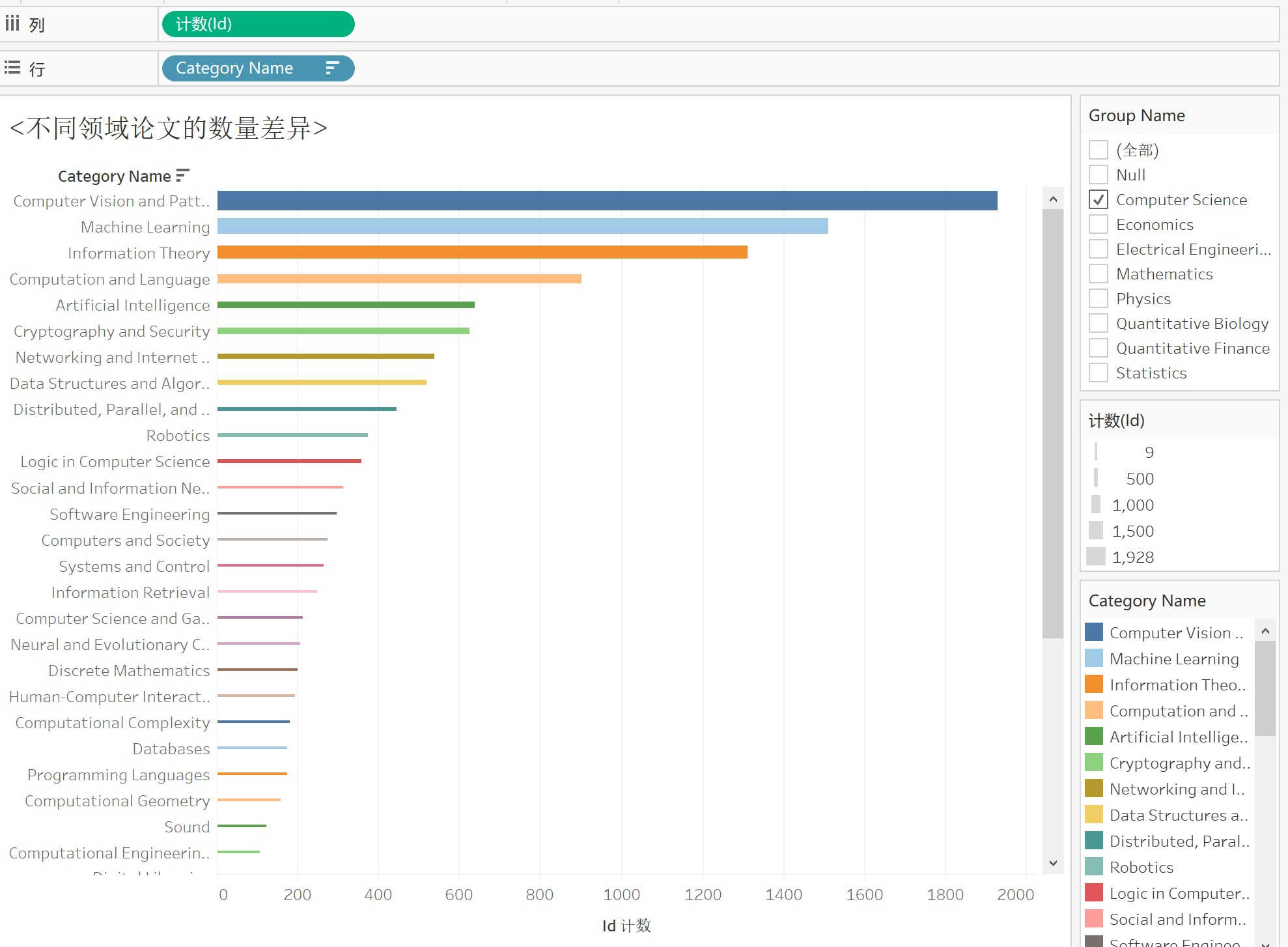4.2计算机领域论文数量变化（top5)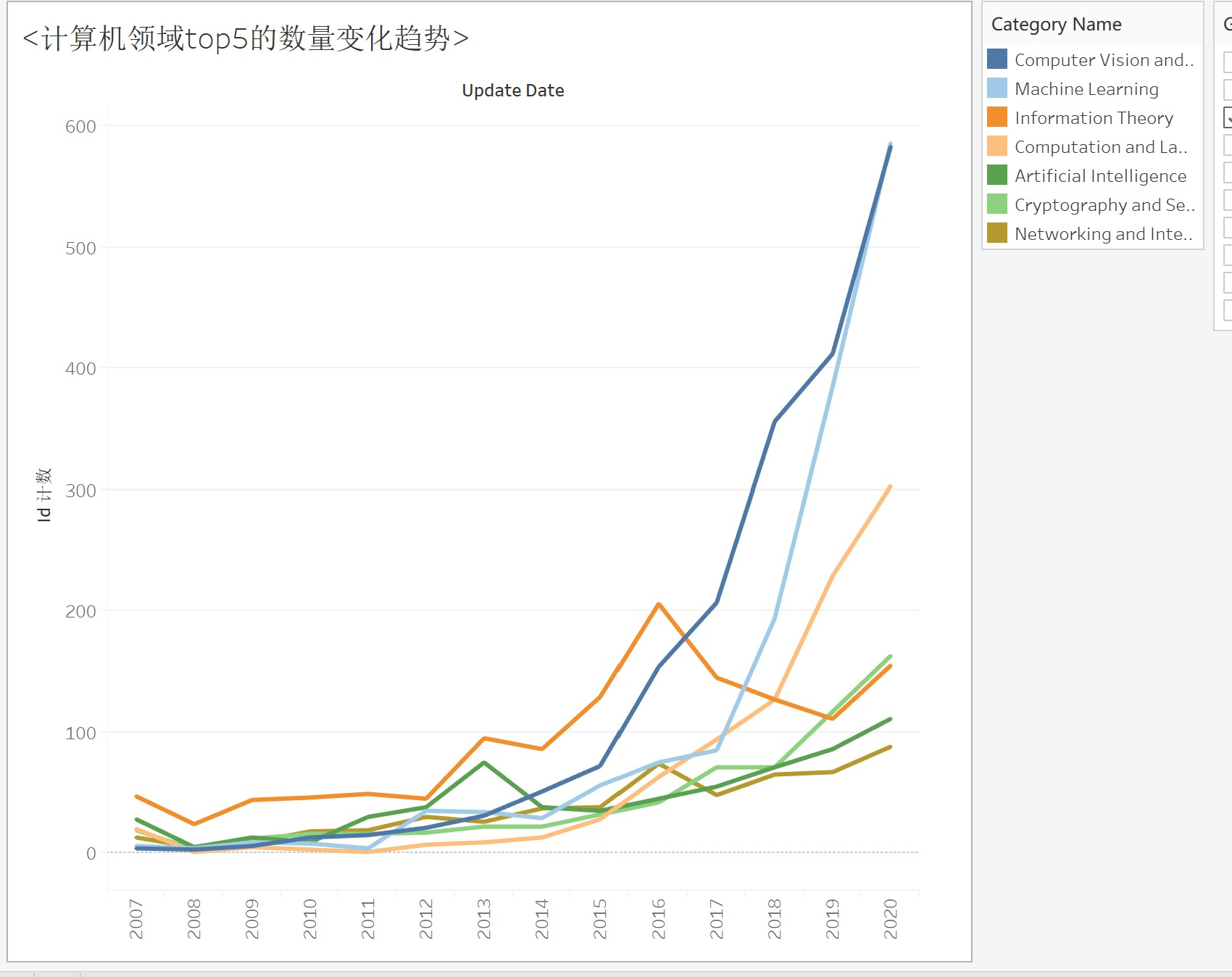4.3计算机领域论文页数差异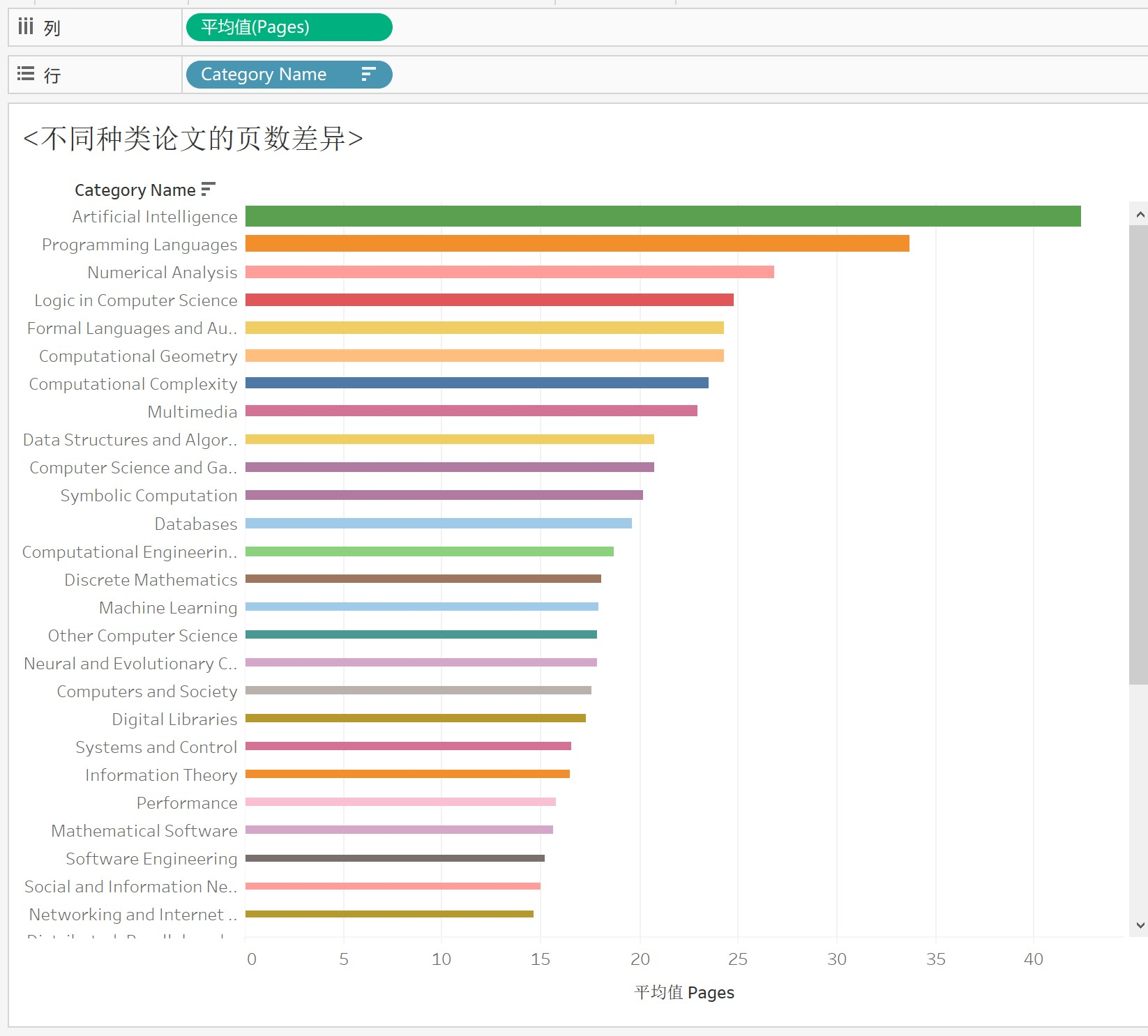总结
总的来说，python，excel，tableau都能完成上述分析可视化任务，但是各有优缺点：

python：

适合做不规则数据的处理
适合进行各种算法建模
适合进行预测分析

excel：

适合小量规则数据的分析与可视化
数据格式较为局限，数据量不易过大

tableau

适合大量数据的分析与可视化
操作简单，比python敲代码作图更快捷


展开全文数据分析 excel 可视化
• 样本熵的计算包和原理见下链接： ...直接上可视化（我看了很多论文的图，都画这样） from sampen import sampen2 def sample_entropy(IImfs): # 计算样本熵 m=1、2, r=0.1、0.2 sampen=[] fo
样本熵的计算包和原理见下链接：

# 计算样本熵 https://sampen.readthedocs.io/en/stable/#with-tox
# 样本熵的解释 https://www.zhihu.com/question/266285555

直接上可视化（我看了很多论文的图，都画这样）

from sampen import sampen2

def sample_entropy(IImfs):
# 计算样本熵 m=1、2, r=0.1、0.2
sampen=[]
for i in IImfs:
for j in (0.1,0.2):
sample_entropy=sampen2(list(i),mm=2,r=j,normalize=True)
sampen.append(sample_entropy)

# 分离
entropy_r1m1=[]  # r=0.1、m=1
entropy_r1m2=[]  # r=0.1、m=2
entropy_r2m1=[]  # r=0.2、m=1
entropy_r2m2=[]  # r=0.2、m=2
for i in range(len(sampen)):
if (i%2)==0: # r = 0.1
# m = 1
entropy_r1m1.append(sampen[i])
# m = 2
entropy_r1m2.append(sampen[i])
else: # r = 0.2
# m = 1
entropy_r2m1.append(sampen[i])
# m = 2
entropy_r2m2.append(sampen[i])

# 可视化
fig=plt.figure()
x=list(range(1,len(IImfs)+1,1))
# 线段、颜色参考：https://blog.csdn.net/qiurisiyu2016/article/details/80187177
plt.plot(x,entropy_r1m1,'r:H',label='m=1 r=0.1')
plt.plot(x,entropy_r2m1,'b:s',label='m=1 r=0.2')
plt.plot(x,entropy_r1m2,'c:D',label='m=2 r=0.1')
plt.plot(x,entropy_r2m2,'m:h',label='m=2 r=0.2')
plt.xlabel('I M F 分 量')
plt.ylabel('样  本  熵  值')
plt.legend()
plt.show()
#fig.savefig('sample_entropy.png', format='png', transparent=True, dpi=300, pad_inches = 0)

m和r都为参数，可在原理链接中看。可自行调整，不过我看论文用的这个。

计算

# 计算各imf分量的样本熵
sample_entropy(IImfs)

结果，此处数据使用上一篇文章中CEEMDAN分解所得的Imf。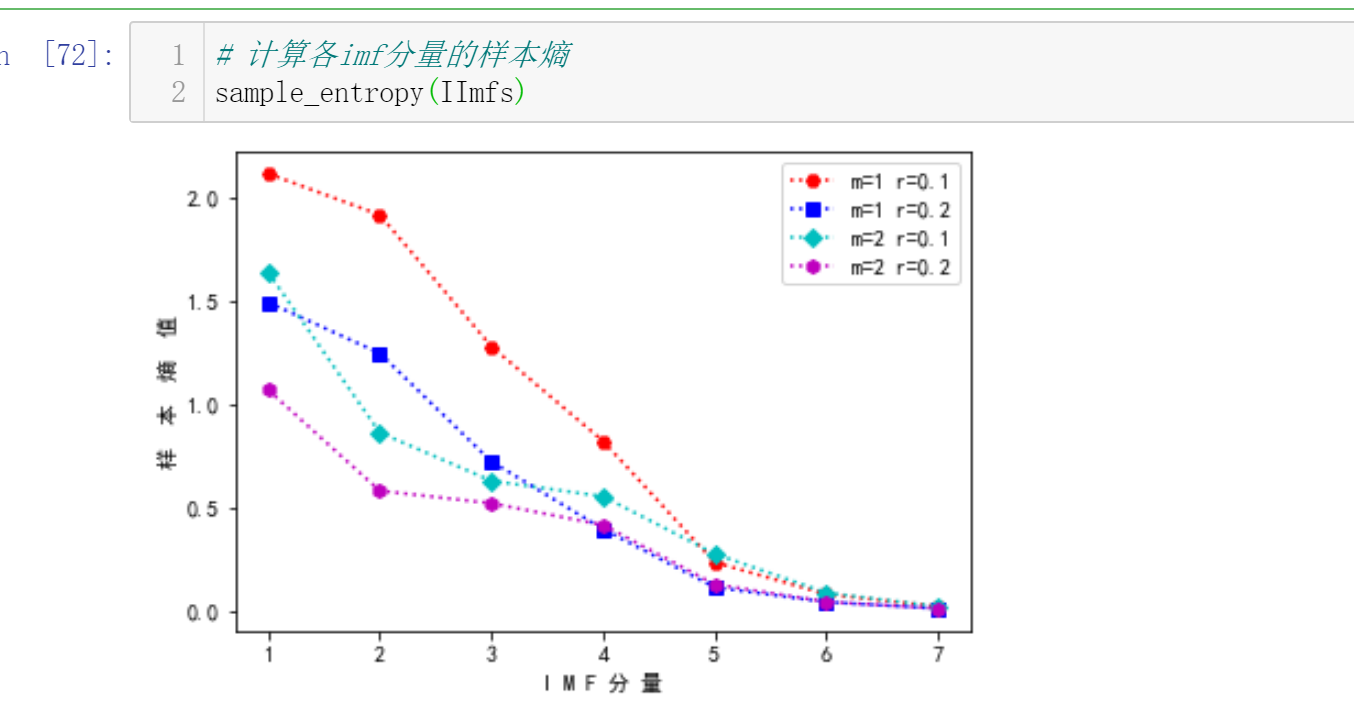展开全文• python recon.py 文件 sample-profile.json：纸上的示例配置文件。 sample-preference.json：纸上的样本偏好。 sample-communication-history.json：每个用户的通信历史。 recon.py：论文实现。
• 入门深度学习和机器学习最重要是兴趣，找到一个做的人少，你可以深入显示...把作者目前有的训练样本分享给大家，供大家训练； 带你解读歌声合成论文； 带你了解合成歌曲的 Python 的模块； 作者歌曲合成样例试听：ht...
入门深度学习和机器学习最重要是兴趣，找到一个做的人少，你可以深入显示你的成果。歌声合成是深度学习一个应用方向，但是它不像图形处理和音频处理，资源很多。需要一个入门老师的引导。GitChat 主要的目标是引导大家入门歌声合成，给予你清晰的实践路线和资料。把作者目前有的训练样本分享给大家，供大家训练；带你解读歌声合成论文；带你了解合成歌曲的 Python 的模块；作者歌曲合成样例试听：https://blog.csdn.net/weixin_32393347/article/details/83353097阅读全文: http://gitbook.cn/gitchat/activity/5d356737fad254490f96b8ce您还可以下载 CSDN 旗下精品原创内容社区 GitChat App ，阅读更多 GitChat 专享技术内容哦。展开全文• 最近基于对抗样本做了一些工作，这里写一篇论文介绍对抗样本基本的原理和生成方法。内容上参考Goodfellow的论文 Explaining and Harnessing Adversarial Examples 一、什么是对抗样本？ 对抗样本的概念最早提出于...
最近基于对抗样本做了一些工作，这里写一篇论文介绍对抗样本基本的原理和生成方法。内容上参考Goodfellow的论文 Explaining and Harnessing Adversarial Examples
目录一、什么是对抗样本？二、对抗样本的线性解释三、快速梯度符号法FGSM生成对抗样本四、资源下载
一、什么是对抗样本？
对抗样本的概念最早提出于2014年Szegedy的论文 Intriguing Properties of Neural Networks. 在论文，作者发现了一种有趣的现象，即：当前流行的机器学习模型包括神经网络会容易以很高的置信度分错和原始样本仅仅有轻微不同的样本，这类样本被称为对抗样本。这一现象揭示了现有机器学习算法的盲点和不足，即没有完全掌握数据的本质特点，从而容易受到被精心设计的对抗样本的攻击
下图很好的描述了对抗样本的作用， 即原始图片在被加上轻微的噪声之后，分类器会以很高的置信度将图片识别为另外一类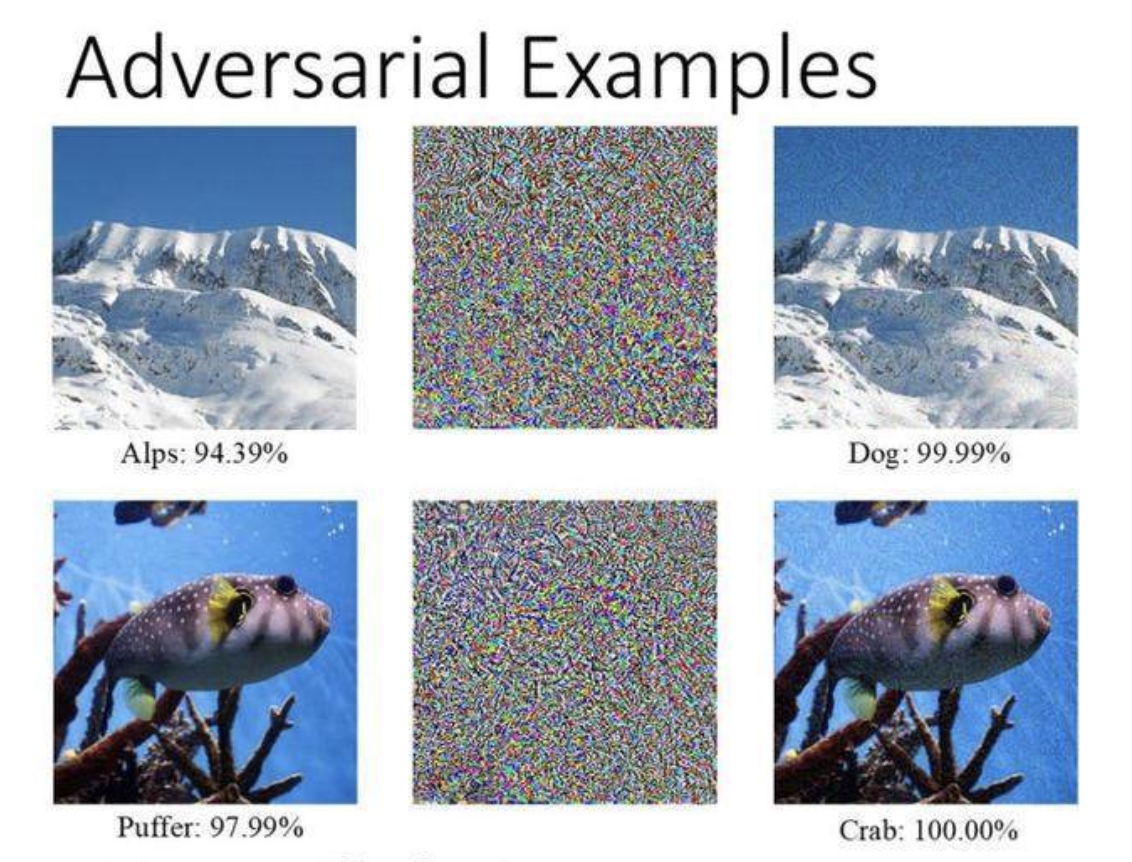那么，对抗样本的原理是什么呢？
二、对抗样本的线性解释
Goodfellow在论文中给出了一个解释：在线性高维情况下，即使是很小的扰动，也会对输出造成非常大的影响。这里将对抗样本记为 $\bar{x}$, 线性模型的权重为$w$, 扰动为$\eta$, 同时扰动的强度限制在一定范围内$\left \|\eta\right \|_L<\varepsilon$. 我们可以有式（1）
$w\bar{x}=wx+x\eta$    （1）
从上式我们可以知道，当$w$的维度很高时，即使扰动$\eta$很小，最终的输出也会受到很大的影响。Goodfellow认为，当前主流的机器学习模型中存在太多的线性性质，如神经网络的每一层其实都是一个线性模型，而常用的ReLu激活函数也局部线性的，所以，在高维情况下，小的扰动也会造成很大的输出误差
三、快速梯度符号法FGSM生成对抗样本
基于提出的线性解释，Goodfellow提出了一种非常简单的生成对抗样本的方法称为fast gradient sign method (FGSM). 式（1）中的扰动$\eta$ 为
$\eta =\varepsilon sign(\bigtriangledown_{x}J(\Theta,x,y))$  (2)
这里$J$表示损失函数，简单来说，就是模型的损失函数对样本求导，再取符号函数，乘上扰动强度，便得到了对抗样本
这里给出一份为普通多层神经网络生成对抗样本的代码
from sklearn.datasets import load_boston
from sklearn import preprocessing
from sklearn.model_selection import train_test_split
from keras import optimizers
import keras.backend as K
from keras import losses

from keras.models import Sequential
from keras.layers import Dense
import warnings

warnings.filterwarnings("ignore")

boston = load_boston()

data = boston.data
data = preprocessing.StandardScaler().fit_transform(data)
label = boston.target
traindata, testdata, trainlabel, testlabel = train_test_split(data,label,test_size=0.2, random_state=0)

model = Sequential()
model.add(Dense(128,input_dim=13,activation='relu'))
model.add(Dense(64,activation='relu'))
model.add(Dense(64,activation='relu'))
model.add(Dense(32,activation='relu'))
model.add(Dense(1))

adam = optimizers.adam(lr=0.01, decay=1e-2)
model.compile(loss='mse',optimizer=adam)

model.fit(traindata,trainlabel,batch_size=64, epochs=200,verbose=0,shuffle=True,validation_split=0.1)
testdata += 0.1
score = model.evaluate(testdata,testlabel,verbose=0,batch_size=64)
print(score)

sess = K.get_session()
testdata_adv = testdata

epochs = 1
epsilon = 0.1

loss = losses.mse(testlabel,model.output)
grads = K.gradients(loss,model.input)

delta = K.sign(grads)
testdata_adv += epsilon*delta

testdata_adv = sess.run(testdata_adv, feed_dict={model.input:testdata})

testdata_adv = preprocessing.StandardScaler().fit_transform(testdata_adv)
score = model.evaluate(testdata_adv,testlabel,verbose=0,batch_size=64)
print(score)


四、资源下载
微信搜索“老和山算法指南”获取下载链接与技术交流群有问题可以私信博主，点赞关注的一般都会回复，一起努力，谢谢支持。


展开全文代码
• 一.论文解读 论文链接：...样本预处理(数据集用celeba，拿来先生成3种尺寸12*12/24*24/48*48，每种尺寸含正、负、部分3类) ——> 写P、R、O网络——> 训练...mtcnn
• 样本测试中实施绘图理论 PDF 绘图 在 apKS 中实现数据类型（REAL 或 INTS）检测 回顾离散数据 (INTS) 处理、源代码和测试 实施 EPL 论文中的实验 创建一个解析命令行输入的主要方法，使其读取 .dat 或 .txt 文件 ...
• 嗨，这是带有深层内核的MMD的pytorch代码，在ICML2020论文“学习用于非参数两样本测试的深层内核”（ICML2020）（ ）中介绍。 软件版本 火炬版本为1.1.0。 Python版本是3.7.3。 CUDA版本是10.1。 大多数代码都需要...
• 基于深度样本的视频着色（Pytorch实现） | | :fire: | 基于深度范例的视频着色，CVPR2019 1,3，1,5，2，1，4， 1，3 1香港科技大学， 2香港城市大学， 3 Microsoft亚洲研究部， 4 Microsoft Cloud＆AI， 5 USC创意...
• 元学习论文总结||小样本学习论文总结 2017-2019年计算机视觉顶会文章收录 AAAI2017-2019 CVPR2017-2019 ECCV2018 ICCV2017-2019 ICLR2017-2019 NIPS2017-2019 使用tqdm 包实现进度条功能 from tqdm import tqdm ...
• 元学习论文总结||小样本学习论文总结 2017-2019年计算机视觉顶会文章收录 AAAI2017-2019 CVPR2017-2019 ECCV2018 ICCV2017-2019 ICLR2017-2019 NIPS2017-2019 作者：路人甲 链接：...
• 我们的论文被爱思唯尔信息科学（IF 5.910）接受 环保环境 OS : Windows10 64bit (We have tested that the code works fine on Ubuntu 18.04) CPU : Intel i5-8500 3.00GHz GPU : Geforce GTX Titan X (Works on GPU...
• ## python日志实现

千次阅读 2018-07-25 14:11:55
元学习论文总结||小样本学习论文总结 2017-2019年计算机视觉顶会文章收录 AAAI2017-2019 CVPR2017-2019 ECCV2018 ICCV2017-2019 ICLR2017-2019 NIPS2017-2019 数据操作完整源码自 vieo.zhu@foxmail.com 申请 ...
• Python代码样本 一种。 大规模计算和本地并行化 包含三个.ipynb分配文件，用于芝加哥大学社会科学的大规模计算课程，举例说明了对PySpark，GPU计算（带有pyopencl ），Slurm批处理作业以及与之结合使用的方法的接触...
• 论文 Belghazi, Mohamed Ishmael, et al. “Mutual information neural estimation.” International Conference on Machine ...NN在维度和样本量上都是线性可伸缩的，MI的计算可以通过反向传播进行训练。 核心机器学习 人工智能 神经网络
• ## python assert in断言

千次阅读 2019-04-18 16:04:04
元学习论文总结||小样本学习论文总结 2017-2019年计算机视觉顶会文章收录 AAAI2017-2019 CVPR2017-2019 ECCV2018 ICCV2017-2019 ICLR2017-2019 NIPS2017-2019 那么我们什么时候应该使用断言呢？如果没有特别的...
• Python脚本和代码以“使用包围锥嵌入的分级图像分类”格式生成和转换图像 参考 描述这个方法能使纸张的arXiv上找到。 可以在找到实现此学习算法的存储库。 再生产 该存储库包含在不同数据集上重现论文结果所需的...
• 代码样本 R和Python代码示例 R样本（拟合幂律分布） 这是项目容忍原则的一部分。 是测试儿童早期话语中单词频率分布的方法。 代码样本中使用的数据是一个孩子... 这是论文： : （在EMNLP 2020的CMCL研讨会上进行）
• 该软件包提供了Python的相似性网络融合（SNF）实现，该技术可将多个数据源组合到一个表示样本关系的图形中。 目录 如果您知道要去哪里，请随时跳转： 要求和安装 此软件包需要Python 3.5或更高版本。 假设您具有正确...
• 则从该总体随机抽取(每抽1例不予返回就抽下1例)含量为n的样本时，其中恰有X例阳性的概率为：式中X的取值是从0与(n-N+M)之较大者开始，直至n与M之较小者为止。又式(1)称为超几何分布的概率函数。在上述条件下，如果每...
• 元学习论文总结||小样本学习论文总结 2017-2019年计算机视觉顶会文章收录 AAAI2017-2019 CVPR2017-2019 ECCV2018 ICCV2017-2019 ICLR2017-2019 NIPS2017-2019 转载：...
• 元学习论文总结||小样本学习论文总结 2017-2019年计算机视觉顶会文章收录 AAAI2017-2019 CVPR2017-2019 ECCV2018 ICCV2017-2019 ICLR2017-2019 NIPS2017-2019 from __future__ import print_function import cv...
• 为什么论文里高达99.8以上的准确率，自己实际使用下来的准确率却并不好，甚至用来落实到百人以内的人脸识别，都经常出现误识别现象，造成这样的现象最主要的原因来自于训练人脸识别模型的样本和国内人脸有差距。...人脸检测 人脸追踪 人脸识别 活体检测 性别年龄识别
• 我们还将一些预处理后的样本数据（包括增强的结果）放入此存储库中。 TMSV的LAVSE使用的数据集（台湾汉语语音与视频）发布。 如果您发现对研究有用的代码，请引用以下论文。 @inproceedings{chuang2020lite, ...
• 元学习论文总结||小样本学习论文总结 2017-2019年计算机视觉顶会文章收录 AAAI2017-2019 CVPR2017-2019 ECCV2018 ICCV2017-2019 ICLR2017-2019 NIPS2017-2019 python实现relu、sigmoid、阶跃函数 # coding:utf-8...
• 这是AAAI 2019论文“”的实验代码。 如果您有任何问题，请随时与作者，和。 抽象的 生存分析是统计研究中使用数据审查处理为事件到时间信息建模的一个热点，它已广泛用于许多应用中，例如临床研究，信息系统和存在...
• 元学习论文总结||小样本学习论文总结 2017-2019年计算机视觉顶会文章收录 AAAI2017-2019 CVPR2017-2019 ECCV2018 ICCV2017-2019 ICLR2017-2019 NIPS2017-2019 Opencv之图像分割原理：...
• 跨尺度非局部注意和穷举自样本挖掘的图像超分辨率 该存储库适用于以下论文中介绍的CSNLN ，，，，，和，“影像超分辨率与跨尺度非本地关注和详尽的自我模范矿业”，CVPR2020， 该代码基于构建，并在带有Titan / Xp...
• ## windows7 + python3.6 + TensorFlow实现FCN

千次阅读 热门讨论 2018-04-25 13:18:18
随记：新的工作开始研究基于深度学习的图像分割了，在入职之前自己先看了一下图像分割方面的论文和视频，在自己动手训练样本的时候，由于刚开始接触python 和TensorFlow，以至于出现了很多小问题，如今相关的工程...TensorFlow FCN
• 元学习论文总结||小样本学习论文总结 2017-2019年计算机视觉顶会文章收录 AAAI2017-2019 CVPR2017-2019 ECCV2018 ICCV2017-2019 ICLR2017-2019 NIPS2017-2019 参考：......

# python论文样本python 订阅